# Electron States and Light Absorption in Strongly Oblate and Strongly Prolate Ellipsoidal Quantum Dots in Presence of Electrical and Magnetic Fields

## Abstract

In framework of the adiabatic approximation the energy states of electron as well as direct light absorption are investigated in strongly oblate and strongly prolate ellipsoidal quantum dots (QDs) at presence of electric and magnetic fields. Analytical expressions for particle energy spectrum are obtained. The dependence of energy levels’ configuration on QD geometrical parameters and field intensities is analytically obtained. The energy levels of electrons are shown to be equidistant both for strongly oblate and prolate QDs. The effect of the external fields on direct light absorption of a QD was investigated. The dependence of the absorption edge on geometrical parameters of QDs and intensities of the electric and magnetic fields is obtained. Selection rules are obtained at presence as well as absence of external electric and magnetic fields. In particular, it is shown that the presence of the electric field cancels the quantum numbers selection rules at the field direction, whereas in radial direction the selection rules are preserved. Perspectives of practical applications for device manufacturing based on ellipsoidal quantum dots are outlined.

## Introduction

Recent interest to semiconductor quantum dots (QDs) is conditioned by new physical properties of these zero-dimensional objects, which are conditioned by size-quantization (SQ) effect of the charge carriers (CCs) . Such new structures were obtained by means of interrupted growth of QDs within semiconductor media. Development of new growth technologies, in particular such as Stranski-Krastanov epitaxial method, made possible development of QDs having various shapes and dimensions. It is known that energy spectrum of CCs of a QD is fully quantized and similar to the energy spectra of atoms, in view of that the QDs are often called “artificial atoms” . Most of the research in this area is focused on studies of the spherical QDs. However during last years the ellipsoidal, pyramidal, cylindrical and lens-shaped QDs are also undergoing theoretical and experimental investigations . Due to presence of strong SQ effect in the mentioned objects the physical characteristics of CCs in such systems strongly depend on the geometrical shapes of QDs. Even slight variation of the shapes significantly affects the CC spectrum [11, 12]. In other words, QD geometric shapes and dimensions may serve as useful tools for CC energy spectrum and other characteristic parameters variation inside a QD for various practical applications in systems comprised of QD ensembles. Monitored ‘shaping’ during the process of growth makes possible simulation and development of samples with desired physical parameters.

Another powerful factor affecting the CC energy spectrum shaping inside QD is the confinement potential of the QD—Media Interface front of the growth. The semiconductor nanostructures based on GaAs/Ga 1−x Al x As-type systems are objects of intensive recent investigations due to wide band gap and availability of well elaborated growth techniques of various systems incorporating such materials. As a result of natural diffusion process during the growth of QDs, the correspondently forming confinement potential is such that can be easily approximated in most cases by a parabolic potential. Also note, that for this approximation the Kohn theorem is well generalized, this proves that such approximation is correct, the experimental verification is provided in Ref. . However, the effective parabolic potential may origin due to peculiarity of the QDs shape . Such realization is possible for strongly oblate (or prolate) QDs shape. Besides, the rotational ellipsoids, or spheroids, in contrary to spheres, are known to be described by two parameters (short and large half-axes instead of radius). In addition to that the external electric and magnetic fields causing quantization are alternative tools of control of the energy spectrum of QDs CC. The strong external fields, at certain values of their intensities, may have the same, or even stronger SQ effect on the energy spectrum than the quantum dot’s shape variation. Note, that the magnetic field affects the CC motion only in transversal direction, in difference to the electrical field. Therefore two fields directed in parallel open possibility for a broad manipulation of the CC characteristics inside semiconductor SQ systems.

In particular in paper  the quantum effect of the magnetic field inside the of the strongly prolate QD is investigated. The effect of electrical field on the CC energy spectrum inside the mentioned system has been considered in paper . However, the combined effect of unidirectional electric and magnetic fields is not considered yet.

Analysis of the optical absorption spectra of various semiconductor structures represents a powerful tool for obtaining numerous characteristics of these structures, namely: forbidden gap widths, effective masses of electrons and holes, their mobility, dielectric features, etc. Many papers study these spectra by experiments and analysis, both in massive and SQ semiconductor structures (see e.g. ). SQ phenomenon strongly affects the character of absorption. Indeed, presence of new SQ energy levels makes possible to realize new inter-band transitions widening the scope of applications of devices based on such systems. Meanwhile existence of the external quantizing fields often results in restructuring of the energy levels, as well as creation of new selection rules during the process of the light absorption. Therefore electronic states and direct inter-band light absorption are considered below for strongly oblate ellipsoidal quantum dots (SOEQD) and strongly prolate ellipsoidal quantum dots (SPEQD) at presence of unidirectional electric and magnetic fields; the problem is considered for strong SQ regime.

## Theory

### SOEQD Case: Electronic States Inside the Strongly Oblate Ellipsoidal Quantum Dot in the Presence of Unidirectional Electric and Magnetic Fields

Let us to consider an impenetrable SOEQD located in unidirectional electric and magnetic fields (see Fig. 1a). The potential energy of a charged particle (electron, or hole) in such structure has the following form: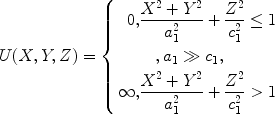(1)

where a 1 and c 1 are the short and long semiaxes of SPEQD, respectively.

As is known, in the strong SQ regime, the energy of Coulomb interaction between electron and hole can be considered much smaller than the energy created by the SOEQD walls. In the framework of such approximation, one can neglect the electron–hole interaction energy. Thus, the problem is reduced to analytical determination of the energy separate expressions for electron and hole (as for non-interacting particles). The quantum dot shape indicates that particle motion along the Z-axis takes place faster than in the normal direction, this also allows to utilize adiabatic approximation. The system Hamiltonian under these conditions has the following form:(2)

in which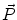is the particle momentum operator,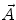is the vector potential of the magnetic field,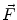is the electrical field intensity,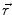is the radius-vector, s is the light velocity in vacuum, and e is the magnitude of electron charge.

Assuming the calibration of vector potential in cylindrical coordinates to have a form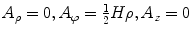, one can express the system Hamiltonian as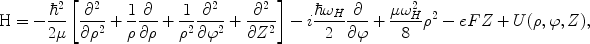(3)

which may be represented as a sum of two Hamiltonians of “fast”H 1 and “slow”H 2 subsystems in dimensionless variables: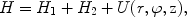(4)

where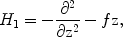(5)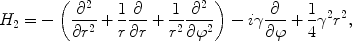(6)

with assumed notations: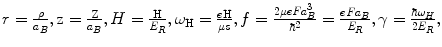where μ is the effective mass of electron,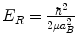is effective Rydberg energy,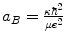is the effective Bohr radius of electron, and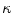is the dielectric permeability. The homogenous electrical and magnetic fields are given by relations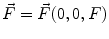and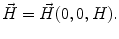Here we assume the wave function (WF) to have the following form: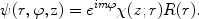(7)

Following the above-mentioned adiabatic approximation, when the coordinater of the “slow” subsystem is fixed, the particle motion is localized in one-dimensional potential well having effective width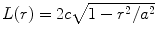, wherea = a 1/a B andc = c 1/a B .

The Schrödinger equation for the “fast” subsystem will acquire the following form: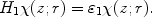(8)

After a number of simple transformations and numerical simulation, for the close-to-bottom energy levels of the spectrum (when the particle is predominantly localized in the regionr a) one can obtain the following power series expression with high degree of accuracy: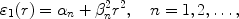(9)

where α n and β n parameters depend on the value of the electrical field. The relation (9) represents an effective potential which is incorporated in the “slow” system Schrödinger equation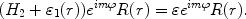(10)

Thus by solving the Eq. 10), we shall obtain the ultimate energy relation for the charged particle (electron, hole)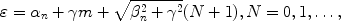(11)

where N = 2n r + |m|,n r and m are the radial and magnetic quantum numbers, respectively.

### SPEQD Case: Electronic States Inside a Strongly Prolate Ellipsoidal Quantum Dot in the Presence of Unidirectional Electric and Magnetic Fields

Now let us to consider an impenetrable SPEQD located in the unidirectional electric and magnetic fields (see Fig. 1b). For this case the potential energy of the charged particle (electron, or hole) will have the form (1) under the conditiona 1c 1, wherea 1andc 1are the short and long semiaxes of SPEQD, respectively. As in the SOEQD case, in the strong SQ regime we neglect the Coulomb interaction between the electron and hole. The shape of QD depicted in the Fig. 1b makes possible the particle motion in the radial plane to be faster than along the Z-axis, which also allows to use adiabatic approximation just like in the SOEQD case. Here the system Hamiltonian has the form (4), where instead of Eqs. 5and6, for the SPEQD case we have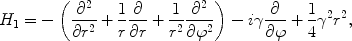(12)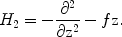(13)

The homogenous electrical and magnetic fields are represented asand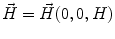just as is in the previous case, while the vector potential is. For this case we assume the WF to have a structure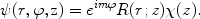(14)

Following the above-mentioned adiabatic approximation, when thez coordinate of the “slow” subsystem is fixed, the particle motion is localized in two-dimensional potential well having effective width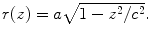The Schrödinger equation for the “fast” subsystem has the form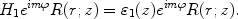(15)

After number of transformations we shall obtain the following energy expression ɛ1(z) for the “fast” sub-system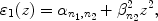(16)

where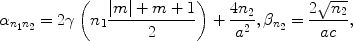(17)

while n 1 and n 2 are some numbers depending on the magnetic field intensity. The expression (16) is an effective potential being incorporated in the Schrödinger equation for the “slow” subsystem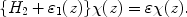(18)

Solution of the Eq. 18gives the final energy expression for the charged particle (electron, hole)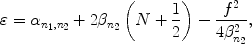(19)

### Direct Inter-Band Light Absorption

Another notable issue of the problem is the direct inter-band absorption of the light by SOEQD in the strong SQ regime. In other words, the relationc {a B e,a B h} holds, wherea B e(h)is the effective Bohr radius of electron (or hole). We consider the case of a “heavy” hole, when μ e μ h , μ e and μ h are the effective masses of electron and hole, respectively.

The absorption coefficient is given by the expression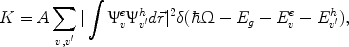(20)

where v and v′ are sets of quantum numbers (QN) corresponding to the electron and heavy hole, E g is the forbidden gap width in the bulk semiconductor, Ω is the incident light frequency and A is a quantity proportional to the square of matrix element in decomposition over Bloch functions.

Numerical calculations were made for the QD fromGaAs, with parameters: μ e = 0.067m e , μ e = 0,12μ h ,E R = 5.275 meV,a B e = 104 Å,E g = 1.43 eV. Finally, for the quantityK and for the absorption edge (AE) we have obtained: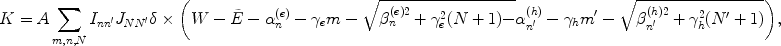(21)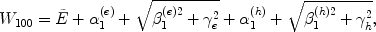(22)

where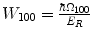, while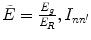and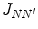are certain integral quantities.

Let us now to consider the selection rules in this case. In the absence of the fields, the transitions between the levels with QNN = N′,n = n′ andm = −m′ are allowed. When the external fields are present the transitions are possible in all cases of magnetic quantization in the radial direction, between the levels with magnetic QNsm = −m′. Under weak magnetic quantization, whena H a 1, where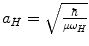is magnetic length, transitions are allowed between the levels with radial QNsn r = n r , and thus between the oscillatory QNsN = N′. In the case whena H a 1, the selection rules among the oscillatory QNs are cancelled, which is a result of competition between size and magnetic quantization. A magnetic field of extremely high-intensity, for whicha H a 1, restores the selection rules between the oscillatory QNs. It should be also noted that the selection rules in the direction of electrical field are becoming obsolete.

Consider now a direct inter-band light absorption inside SPEQDs in the strong SQ regime. The absorption coefficient is given by the relation (20). In this particular case for theK and AE we obtained the following expressions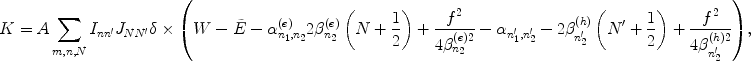(23)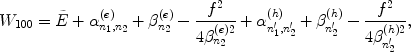(24)

respectively; where, while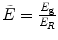, where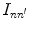andare certain integral quantities.

At absence of the fields at the SPEQD case, the transitions between the QNsN = N′,n r = n r , andm = −m′ are allowed. The application of the external fields to the SPEQD the following alternations from the previous case can be indicated. When the magnetic quantization is either weak or moderate, i.e. whena H a 1ora H a 1(herea 1is the short half-axis), the selection rules in the radial direction are becoming completely obsolete. As a result of this, the selection rules between the oscillatory QNs are also eliminated. Meanwhile, transitions between the magnetic QNs remain unchanged:m = −m′. Note that in the hypothetical case of extremely strong magnetic field (whena H a 1) the selection rules for transitions between the oscillatory QNs are completely restoring.

## Discussion of Results

As one can infer from the obtained results, the CC energy spectrum both of SOEQDs and SPEQDs is equidistant. More correctly, each level of the “fast” subsystem has a family of equidistant “slow” subsystem levels positioned thereupon. The obtained result is valid only for the levels close to the well bottom (or having low QN values), due to the assumed adiabatic approximation.

Note that CC energy levels are equidistant in the absence of external fields. However, the transition frequency between the equidistant levels when the fields are present is usually higher. For example, in the absence of fields, when half-axes of SOEQD are a 1 = 2.5a B c 1 = 0.5a B , the transition frequency for the first equidistant family is obtained equal towhich falls into the IR part of spectrum. With the same half-axes, but in the presence of fields H = 10 T, F = 500 V/cm the transition frequency is almost one-and-half times higher: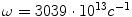. The only exception is SPEQD with H = 0, F ≠ 0. In that case all equidistant levels are shifted for the same value depending on the intensity of the electrical field. The inter-level distance remains the same as in the absence of electrical field (shifted oscillator ).

Figures 2 and 3 illustrate the dependence of CC energy spectrum families inside SOEQD on intensities respectively of electrical and magnetic fields, under fixed lengths of half-axes. One can see from Fig. 2 that the first family of the CC energy spectrum falls with growth of the electric field intensity, while the second family grows and then reduces . This behavior was called “anomalous Stark effect” by the authors of Ref. . They explained such field dependence of energy levels by behavior of the particle probability density |Ψ|2. However, no such anomaly is revealed under correct definition of the Stark effect. In other words, the dependence of the inter-level distance on the electrical field intensity has monotonously growing character. Thus the Stark effect in the described experiments has normal character.

As one can see from the Fig. 3, all levels of the CC energy spectrum family are growing when the magnetic field intensity is increased. This is conditioned by growth of the magnetic quantization contribution into the CC energy increase. Inter level distance is increased with magnetic field intensity while the levels are remaining equidistant.

Figures 4 and 5 show the dependence of AE inside SOEQD on the intensities of electrical and magnetic fields, respectively, when the lengths of SOEQD half-axis are fixed. One can see from Fig. 4 that AE value is shifted towards longer wavelengths when the electrical field intensity is growing. This fact is explained by a change of the quantum-well bottom caused by the external electrical field. As a result of this phenomenon, the energy of electron is reduced, while the energy of hole increases, which in turn reduces the forbidden gap width . The inverse character of the behavior of the AE is illustrated in Fig. 5. Presence of the magnetic field increases the energy of the both particles, independently of the charge sign. Otherwise, the AE shift is due to magnetic quantization effect (blue shift).

Figure 6 illustrates the three-dimensional view of the ground state energy for a CC inside the SPEQD as a function of electrical and magnetic field intensities under fixed lengths of half-axis. One can see that the effects of the electrical and magnetic fields are different: increase of the magnetic field intensity increases the particle energy, while increase of the electrical field intensity reduces the particle energy. The first phenomenon is explained by a growth of the magnetic quantization contribution into CC energy increase, while the second by a change of potential well bottom, or competition of SQ and electrical fields effect on CC.

Similar dependence of AE on the electrical and magnetic fields in SOEQD is illustrated in Fig. 7. The behavior of this dependence can be explained similarly as in the case of SPEQD.

So far we have studied the absorption of a system consisting of semiconductor QDs having identical dimensions. For comparison of the obtained results with experimental data, one has to take into account the random character of SPEQD and SOEQD dimensions (or half-axis) obtained during the QD technological growth process. So, for making the comparison the absorption coefficient should be multiplied by concentration of QDs. Then, instead of the distinct absorption lines we will obtain a series of fuzzy maximums as a result of the size dispersion by semiaxis. For illustration of the size dispersion by the semiaxis we used two experimentally observable models. In the first model we used the Lifshits-Slezov distribution function :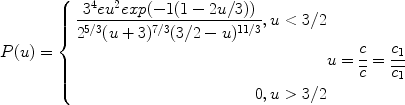(25)

where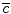is some average value of the semiaxis. In the second model the Gaussian distribution function is used (see e.g. ):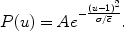(26)

Figure 8 illustrates the dependence of the absorption coefficient K  on the frequency of incident light, for the ensemble of SOEQDs in the absence of external fields. Note that in the model of Gaussian dots distribution, a single distinctly explicit maximum of absorption is observed. When the light frequency is increasing, the second slightly notable maximum is traced. Further increase of the incident light frequency results in decrease of the absorption coefficient. When the Lifshits-Slezov model is realized during a device growth, a number of distinctly explicit absorption maximums as a function of incident light frequency is observed. Note that weakly expressed oscillations on the first peak are seen in the second case, which are due to inter-band transitions of the first equidistant family (see additional graphical insertion in Fig. 8).

## Outline of Possible Practical Applications

Ellipsoidal QDs, especially SOEQDs or SPEQDs, have various commercial applications, in particular in large two-dimensional focal plane arrays in the mid- and far infrared (M&FIR) region, having important applications in the fields of pollution detection, thermal imaging object location and remote sensing as well as IR imaging of astronomical objects (see e.g. US Patent # 6541788).

## References

1. Harrison P: Quantum Wells, Wires and Dots: Theoretical and Computational Physics. John Wiley & Sons Ltd, NY; 2005.

2. Bastard G: Wave Mechanics Applied to Semiconductor Heterostructures. Les editions de physique, Les Ulis Cedex, Paris; 1989.

3. Kazaryan EM, Petrosyan SG: Basic Physics of Semiconductor Nanoelectronics. Russian-Armenian University Publishing, Yerevan; 2005.

4. Bayer M, Stern O, Hawrylak P, Fafard S, Forchel A: Nature. 2000, 405: 923. COI number [1:CAS:528:DC%2BD3cXks1Wmu7o%3D] COI number [1:CAS:528:DC%2BD3cXks1Wmu7o%3D] 10.1038/35016020

5. K.G. Dvoyan, in Proceedings of Semiconductor Micro_and Nanoelectronics the Fifth International Conference (Agveran, Armenia, September 16–18, 2005), p. 165

6. Boze C, Sarkar CK: Physica B. 1998, 253: 238. 10.1016/S0921-4526(98)00407-4

7. Califano M, Harrison P: J. Appl. Phys.. 1999, 86: 5054. COI number [1:CAS:528:DyaK1MXms1eqsr0%3D] COI number [1:CAS:528:DyaK1MXms1eqsr0%3D] 10.1063/1.371478

8. Atoyan MS, Kazaryan EM, Sarkisyan HA: Physica E. 2004, 22: 860. COI number [1:CAS:528:DC%2BD2cXjvF2ksbk%3D] COI number [1:CAS:528:DC%2BD2cXjvF2ksbk%3D] 10.1016/j.physe.2003.09.042

9. Hayrapetyan DB, Dvoyan KG: J. Contemp. Phys.. 2005, 40: 365. COI number [1:CAS:528:DC%2BD2MXhtFynur7K] COI number [1:CAS:528:DC%2BD2MXhtFynur7K]

10. D.B. Hayrapetyan, K.G. Dvoyan, in Proceedings of Semiconductor Micro_and Nanoelectronics the Fifth International Conference (Agveran, Armenia, September 16–18, 2005), p. 165

11. Dvoyan KG, Kazaryan EM: Phys. Stat. Sol. (b). 2001,228(3):695. COI number [1:CAS:528:DC%2BD38XhtFKjuw%3D%3D] COI number [1:CAS:528:DC%2BD38XhtFKjuw%3D%3D] 10.1002/1521-3951(200112)228:3<695::AID-PSSB695>3.0.CO;2-P

12. Dvoyan KG, Kazaryan EM, Petrosyan LS: Physica E. 2005, 28: 333.

13. Kazaryan EM, Petrosyan LS, Sarkisyan HA: Physica E. 2000, 8: 19. 10.1016/S1386-9477(00)00124-7

14. Maksym P, Chakraborty T: Phys. Rev. Lett.. 1990, 65: 108. COI number [1:CAS:528:DyaK3cXltVemu7o%3D] COI number [1:CAS:528:DyaK3cXltVemu7o%3D] 10.1103/PhysRevLett.65.108

15. K.G. Dvoyan, in Proceedings of Semiconductor Micro_and Nanoelectronics the Fourth National Conference (Tsakhckadzor, Armenia, May 29–31, 2003) pp. 55–58

16. Efros AlL, Efros AL: Semiconductors. 1982, 16: 772.

17. Cusack MA, Briddon PR, Jaros M: Physica B. 1998, 253: 10. COI number [1:CAS:528:DyaK1cXlsVyhtL8%3D] COI number [1:CAS:528:DyaK1cXlsVyhtL8%3D] 10.1016/S0921-4526(98)00389-5

18. Banin U, Lee CJ, Guzelian AA, Kadavanich AV, Alivisatos AP: J. Chem. Phys.. 1998, 109: 6. 10.1063/1.476797

19. S. Flugge, Practical Quantum Mechanics, vol. 1 (Mir, Moscow, 1974)

20. Matsuura M, Kamizato T: Phys. Rev. B. 1986, 33: 12. 10.1103/PhysRevB.33.8385

21. Leonard D, Krishnamurthy M, Reaves CM, Denbaars SP, Petroff PM: Appl. Phys. Lett.. 1993, 63: 23. 10.1063/1.110199

22. K.G. Dvoyan, D.B. Hayrapetyan, E.M. Kazaryan, Physica E, in press (2007)

## Acknowledgements

This research has been performed in the framework of the Armenian State Research Program “Semiconductor Nanoelectronics”. The authors express their gratitude to the Rector of Russian-Armenian University, Prof. A.R. Darbinyan, and Vice-Rector on R&D Activity, Prof. P.S. Avetisyan, for administrative and financial support during the research.

## Author information

Authors

### Corresponding author

Correspondence to Karen G Dvoyan.

## Rights and permissions

Reprints and Permissions

Dvoyan, K.G., Hayrapetyan, D.B., Kazaryan, E.M. et al. Electron States and Light Absorption in Strongly Oblate and Strongly Prolate Ellipsoidal Quantum Dots in Presence of Electrical and Magnetic Fields. Nanoscale Res Lett 2, 601 (2007). https://doi.org/10.1007/s11671-007-9079-z